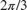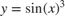# function

Declare function name, inputs, and outputs

## Syntax

function [y1,...,yN] = myfun(x1,...,xM)

## Description

example

function [y1,...,yN] = myfun(x1,...,xM) declares a function named myfun that accepts inputs x1,...,xM and returns outputs y1,...,yN. This declaration statement must be the first executable line of the function. Valid function names begin with an alphabetic character, and can contain letters, numbers, or underscores.You can save your function:In a function file which contains only function definitions. The name of the file must match the name of the first function in the file.In a script file which contains commands and function definitions. Functions must be at the end of the file. Script files cannot have the same name as a function in the file. Functions are supported in scripts in R2016b or later.Files can include multiple local functions or nested functions. For readability, use the end keyword to indicate the end of each function in a file. The end keyword is required when:Any function in the file contains a nested function.The function is a local function within a function file, and any local function in the file uses the end keyword.The function is a local function within a script file.

## Examples

collapse all

Define a function in a file named calculateAverage.m that accepts an input vector, calculates the average of the values, and returns a single result.

function ave = calculateAverage(x) ave = sum(x(:))/numel(x); end

Call the function from the command line.

z = 1:99; ave = calculateAverage(z)
ave = 50

Define a function in a file named stat.m that returns the mean and standard deviation of an input vector.

function [m,s] = stat(x) n = length(x); m = sum(x)/n; s = sqrt(sum((x-m).^2/n)); end

Call the function from the command line.

values = [12.7, 45.4, 98.9, 26.6, 53.1]; [ave,stdev] = stat(values)
ave = 47.3400 stdev = 29.4124

Define a script in a file named integrationScript.m that computes the value of the integrand atand computes the area under the curve from 0 to. Include a local function that defines the integrand,.

Note: Including functions in scripts requires MATLAB® R2016b or later.

% Compute the value of the integrand at 2*pi/3. x = 2*pi/3; y = myIntegrand(x) % Compute the area under the curve from 0 to pi. xmin = 0; xmax = pi; f = @myIntegrand; a = integral(f,xmin,xmax) function y = myIntegrand(x) y = sin(x).^3; end 
y = 0.6495 a = 1.3333 

Define two functions in a file named stat2.m, where the first function calls the second.

function [m,s] = stat2(x) n = length(x); m = avg(x,n); s = sqrt(sum((x-m).^2/n)); end function m = avg(x,n) m = sum(x)/n; end

Function avg is a local function. Local functions are only available to other functions within the same file.

Call function stat2 from the command line.

values = [12.7, 45.4, 98.9, 26.6, 53.1]; [ave,stdev] = stat2(values)
ave = 47.3400 stdev = 29.4124

Define a function that restricts input to a numeric vector that contains no Inf or NaN elements. This function uses the arguments keyword, which is valid for MATLAB® versions R2019b and later.

function [m,s] = stat3(x) arguments x (1,:) {mustBeNumeric, mustBeFinite} end n = length(x); m = avg(x,n); s = sqrt(sum((x-m).^2/n)); end function m = avg(x,n) m = sum(x)/n; end

In the arguments code block, (1,:) indicates that x must be a vector. The validation functions, {mustBeNumeric, mustBeFinite}, restrict the elements in x to numeric values that are not Inf or NaN. For more information, see Function Argument Validation.

Calling the function with a vector that contains an element that is NaN violates the input argument declaration. This violation results in an error being thrown by the mustBeFinite validation function.

values = [12.7, 45.4, 98.9, NaN, 53.1]; [ave,stdev] = stat3(values)
Invalid input argument at position 1. Value must be finite.

## Version History

Introduced before R2006a